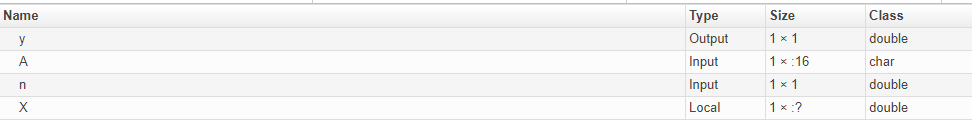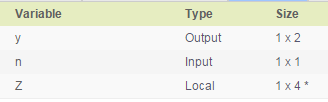Main Content

## MATLAB Language Features Support for GPU Coder

GPU Coder™ supports many of the MATLAB® language features supported by MATLAB Coder™, see MATLAB Language Features Supported for C/C++ Code Generation. However, some features may be supported in a restricted mode and others not supported. In the following sections, we highlight some of the important features that affect GPU code generation and then list the features that not supported by GPU Coder.

A common and important consideration is variable-size matrices support. This feature can really affect the way CUDA® kernels are created and the following discussion describes the feature and considerations for GPU code generation.

### Code Generation for Variable-Size Arrays

For code generation, an array dimension is fixed-size or variable-size. If the code generator can determine the size of an array and that the size of the array does not change at run time, then the dimension is fixed-size. When all dimensions of an array are fixed-size, the array is a fixed-size array. In the following example, `Z` is a fixed-size array.

```function Z = myfcn() Z = zeros(1,4); end```

If the code generator cannot determine the size of an array or the code generator determines that the size changes, then the dimension is variable-size. When at least one of its dimensions is variable-size, an array is a variable-size array.

A variable-size dimension is either bounded or unbounded. A bounded dimension has a fixed upper size. An unbounded dimension does not have a fixed upper size.

In the following example, the second dimension of `Z` is bounded, variable-size. It has an upper bound of 32.

```function s = myfcn(n) if (n > 0) Z = zeros(1,4); else Z = zeros(1,32); end s = length(Z);```

In the following example, if the value of `n` is unknown at compile time, then the second dimension of `Z` is unbounded.

```function s = myfcn(n) Z = rand(1,n); s = sum(Z); end```

You can define variable-size arrays by:

• Using constructors, such as `zeros` or `ones`, with a nonconstant size value

• Assigning multiple, constant sizes to the same variable before using it

• Using loops to grow the dimensions of variables

• Declaring all instances of a variable to be variable-size by using `coder.typeof` or `coder.varsize` functions. For example, `coder.typeof(1, [12,1],[true, false])` and ```coder.varsize(1, [Inf,1], [true, false])```.

For more information, see Define Variable-Size Data for Code Generation.

#### Code Generation Behavior

For variable-size arrays that are bounded, GPU Coder maps these bounded variables to the GPU and CUDA kernels are created. To specify upper bounds for variable-size arrays, see Specify Upper Bounds for Variable-Size Arrays.

For unbounded, variable-size arrays and variable-size arrays whose size is greater than or equal to a `DynamicMemoryAllocation` threshold, GPU Coder does not map these variables to the GPU and kernels are not created. The code generator allocates memory dynamically on the CPU heap. GPU Coder issues a warning for unbounded variables in the build log and code generation report.

By default, the code generator is set to use dynamic memory allocation for variable-size arrays whose size is greater than or equal to the threshold with a threshold value of 2 GB. To change these settings:

• In the configuration object, set the `DynamicMemoryAllocation` to `Threshold` and `DynamicMemoryAllocationThreshold` to a non-negative integer.

• In the GPU Coder app, in the Memory settings, set Dynamic memory allocation to ```For arrays with max size at or above threshold``` and the Dynamic memory allocation threshold to a non-negative integer.

#### Variable-Size Arrays in a Code Generation Report

You can tell whether an array is fixed-size or variable-size by looking at the Size column of the Variables tab in a code generation report.A colon (:) indicates that a dimension is variable-size. A question mark (?) indicates that the size is unbounded. For example, a size of 1-by-:? indicates that the size of the first dimension is fixed-size 1 and the size of the second dimension is unbounded, variable-size. An asterisk (*) indicates that the code generator produced a variable-size array, but the size of the array does not change during execution.### Structure Definition for Code Generation

To generate efficient standalone code for structures, you must define and use structures differently than you normally would when running your code in the MATLAB environment. For code generation, you must first create a scalar template version of the structure before growing it into an array. The code generation inference engine uses the type of this scalar value as the base type of the array. To generate standalone code for MATLAB structures, you are restricted to the following operations:

• Define structures as local and persistent variables by assignment and using the `struct` function

• Index structure fields using dot notation

• Define primary or entry-point function inputs as structures

• Pass structures to local functions

For more information, see Structure Definition for Code Generation.

Note

GPU Coder generates more efficient code when you use struct of arrays instead of array of structs.

#### Example

This example shows how to write a MATLAB function that uses structure arrays so that it is suitable for code generation. First, you must specify the base element using the `struct` function.

```tempS = struct('a',0,'b',0); numE = 2000; AofS = repmat(tempS,numE,1); ```

In MATLAB, when building up a structure array, you would typically add fields as you go. This "dynamic" style of building structures is not supported for code generation. One reason is that it is possible in MATLAB to have different structure fields for two different elements of a structure array, which conflicts with the more static approach of type inference. Therefore, you must specify the base scalar element first, and then grow a structure array from this fully specified element. This method guarantees that two elements of a structure array always share type (fields).

```for ind = 1:numE AofS(ind).a = rand; AofS(ind).b = rand; end ```

Now, you can define an entry-point function `mStructSupport` that takes `AofS` as input. The local function `arrayOp` doubles `AofS.b` and stores the result in `AofS.a`.

```function [V] = mStructSupport(AofS) V = arrayOp(AofS); end function AofS = arrayOp(AofS) n = numel(AofS); for i = 1:n AofS(i).a = AofS(i).b * 2; end end```

You can use any of the methods described in Code Generation by Using the GPU Coder App to generate CUDA code for this example.

### Unsupported Features

The following list contains the features that are not currently supported.

• Memory integrity checks, see Control Run-Time Checks.

• Array bound and dimension checks.

• `break` statements.

• Function handles are supported only when defined within another function and not as entry-point parameter.

• Anonymous functions are supported only when defined within another function and not as an entry-point parameter.

• MATLAB classes.

## Support

#### Generating CUDA Code from MATLAB: Accelerating Embedded Vision and Deep Learning Algorithms on GPUs

Download white paper